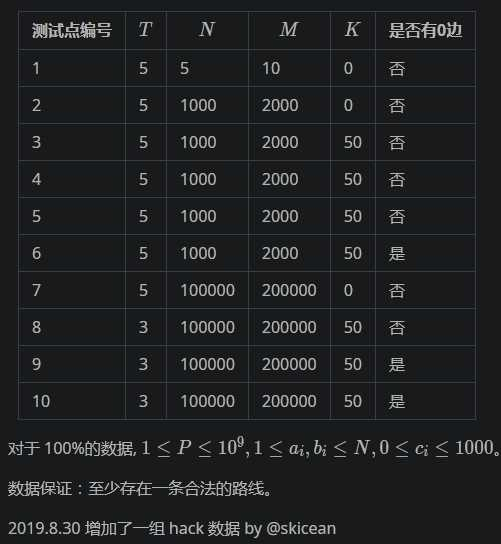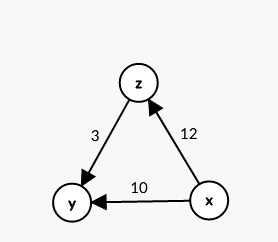# P3953 逛公园

2
5 7 2 10
1 2 1
2 4 0
4 5 2
2 3 2
3 4 1
3 5 2
1 5 3
2 2 0 10
1 2 0
2 1 0

3
-1

## 说明/提示

【样例解释1】

【测试数据与约定】x=dis[p]+w-dis[now]+k

dp方程就是

f[p][x]=(f[p][x]+f[now][k]) mod p

## 代码

``````#include<iostream>
#include<cstdio>
#include<algorithm>
#include<cstring>
#include<queue>
using namespace std;
const int N = 1e5+10;
int n,m,T,k,p,tot = 0,u,v,w,sum = 0;
bool vis[N],in[N];
struct node{int to,net,w;}e,edge;
priority_queue<pair<int,int>, vector<pair<int,int> >, greater< pair<int,int> > >q;
{
int s = 0,w = 1; char ch = getchar();
while(ch < ‘0‘ || ch > ‘9‘){if(ch == ‘-‘) w = -1; ch = getchar();}
while(ch >= ‘0‘ && ch <= ‘9‘){s = s * 10+ch -‘0‘; ch = getchar();}
return s * w;
}
{
e[++tot].w = w;
e[tot].to = y;
edge[++sum].w = w;
edge[sum].to = x;
edge[sum].net = hed[y];
hed[y] = sum;
}
void chushihua()
{
tot = 0, sum = 0;
memset(vis,0,sizeof(vis));
memset(f,0,sizeof(f));
memset(hed,0,sizeof(hed));
memset(dis,0x3f3f,sizeof(dis));
memset(in,0,sizeof(in));
}
void dij()//在反向图上跑最短路
{
q.push(make_pair(0,n)); dis[n] = 0;
while(!q.empty())
{
int t = q.top().second; q.pop();
if(in[t]) continue;
in[t] = 1;
for(int i = hed[t]; i; i = edge[i].net)
{
int to = edge[i].to;
if(dis[to] > dis[t] + edge[i].w)
{
dis[to] = dis[t] + edge[i].w;
q.push(make_pair(dis[to],to));
}
}
}
}
int dfs(int x,int rest)//记忆化搜索，rest表示当前还能比最短路多走的距离
{
if(vis[x][rest]) return -1; //判0环
if(f[x][rest]) return f[x][rest];//记忆化搜索
if(x == n) f[x][rest] = 1;//到达n点，说明找到一条可行的方案数
vis[x][rest] = 1;//打个标记
for(int i = head[x]; i; i = e[i].net)
{
int to = e[i].to;
int tmp = dis[to] + e[i].w - dis[x];//计算走这条边要比走最短路多经过的距离
if(rest - tmp >= 0)//后面还能再接上
{
int now = dfs(to,rest-tmp);//往下搜
if(now == -1)
{
return f[x][rest] = -1;
}
f[x][rest] =(f[x][rest] + now) % p;//f[x][rest]表示从x到n还能比最短路多走rest的方案数
}
}
vis[x][rest] = 0;
return f[x][rest]%p;
}
int main()
{
while(T--)
{
chushihua();
for(int i = 1; i <= m; i++)
{
}
dij();
printf("%d\n",dfs(1,k));
}
return 0;
}
``````

### ENDING

P3953 逛公园

(0)
(0)